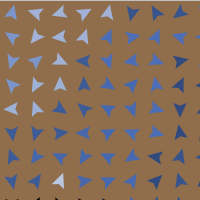# Axelrod_Cultural_Dissemination (version 1.1.0)

MODEL PURPOSE:

The Axelrod’s model of cultural dissemination is an agent-model designed to investigate the dissemination of culture among interacting agents on a society.

MODEL DESIGN:

Axelrod model consists in a population of agents, each one occupying a single node of a square network of size L. There are L^2 agents. The culture of an agent is described by a vector of F integer variables called features. Each feature consists in a nominal variable that can assume q different values (called traits) between 0 and q - 1. In the original Axelrod’s model the interaction topology is regular bounded (non-toroidal), each agent can interact only with its four neighbors (von Neumann neighborhood) and agents can not move.

In the present implementation of the model using NetLogo each agent is located at each patch of the grid with the default agent shape. We have set toroidal boundaries (but the simulation can properly function as well in the original non-toroidal one). We have included a parameter radius which can take positive real values o explore the implications of other neighborhood sizes. The neighborhood agent set is obtained including all neighbors at distance less or equal than radius where radius is used as a distance from the agent own coordinates. Then, the four von Neumann neighbors are at distance radius = 1 when an agent is exactly in the center of its patch.

It is also implemented the possiblity for agents to move according to three parameters: veloc, steplength and angle. If moving, select the velocity of agent movement with veloc greater than zero, select the length of the step with steplength and the angle of rotating with angle. At each time step agents decide to move taking veloc as a probability. In case of actual movement, agents select at random a value between zero and angle. If the value is in the upper half of angle it is added (as and angle) to the current direction of the agent. If it is in the lower half it is subtracted. Ones a direction is selected, agent moves a distance steplength.

For visualization, each agent adopts a color representing its culture. This is done taking the agent culture traits as a number, computing the corresponding number of base q and mapping this number to a color number in the blue range between 100 and 109.9.### Release Notes

We included an extensions of Axelrod’s model: agents can move.
Then, you should also decide if the agents can move or not. In original Axelrod model the agents do not move. If moving, select the velocity of agent movement with ‘veloc’, select the length of the step with ‘steplength’ and the angle of rotating with ‘angle’. At each tick agents decide to move taking ‘veloc’ as a probability. In case of actual movement, agents select at random a value between 0 an ‘angle’. If this values is in the upper half, then it is added as an angle to the current direction of the agent. If it is in the lower half it is subtracted. Then, ones direction is selected, agent moves a distance ‘steplength’.

Here we have setted toroidal boundaries, but the simulation can properly function as well in the original non-toroidal one. In our case, the four von Neumann neighbors are at distance ‘radius’ one. The model permits to change the value of ‘radius’ to explore the implications of other neighborhood sizes.

At the end, in the absorbing final state, when calculating for the number of regions, the model makes different visible networks which include all neighbors agents with the same culture. Then, when counting the number of cultural domains it is considered that two domains are different if they are not connected, even if agents in both domains share same culture.

Note also that two agents could have similar (but with zero overlap) cultural values and then, its corresponding colors could be so similar that it could induce to think that the cultural values are the same. Just check to see that it is not.